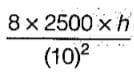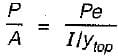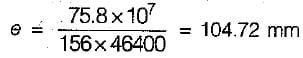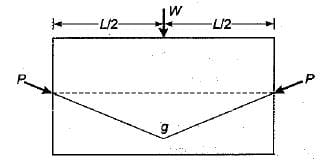# Test: Prestress Concrete - 2

## 10 Questions MCQ Test Topicwise Question Bank for Civil Engineering | Test: Prestress Concrete - 2

Description
Attempt Test: Prestress Concrete - 2 | 10 questions in 30 minutes | Mock test for Civil Engineering (CE) preparation | Free important questions MCQ to study Topicwise Question Bank for Civil Engineering for Civil Engineering (CE) Exam | Download free PDF with solutions
QUESTION: 1

Solution:
QUESTION: 2

Solution:
QUESTION: 3

### If a simply supported concrete beam, prestressed with a force of 2500 kN, is designed by load balancing concept for an effective span of 10 m and to carry a total load Of 40 kN/m, the central dip of the cable profile should be

Solution:

Let the dip of the cable be h.
Upward pressure provided by the parabolic cable = 8Ph/L2
8Ph/L2 = w
⇒= 40  ⇒ h = 0.2 m
⇒ h = 200 mm

QUESTION: 4

A prestressed concrete beam has a cross- section with the following properties:
A = 46,400 mm2, I = 75.8 x 107 mm4,
ybottom = 244 mm, ytop = 156 mm.
It is subjected to a prestressing force at an eccentricity ‘e’ so as to have a zero stress at the top fibre. The value of ‘e’ is given by

Solution:

For zero stress at top fibre,
P/A = Pe/Z
⇒⇒QUESTION: 5

in pre-stressed concrete

Solution:
QUESTION: 6

The purpose of reinforcement in pre-stressed concrete is

Solution:
QUESTION: 7

Prestress loss due to friction occurs

Solution:
QUESTION: 8

The coefficient of shrinkage for high grade concrete for pre-tensioned work is

Solution:
QUESTION: 9

If 'P' is the prestressing force applied at a maximum eccentricity 'g' at mid-span, figure, to balance the concentrated load 'W', the balancing load will beSolution:
QUESTION: 10

Consider the following factors
1. initial prestress
2. losses in prestress
3. depth of cable from extreme compression fibre
Those which affect the ultimate moment capacity of a prestressed concrete beam would include

Solution:Use Code STAYHOME200 and get INR 200 additional OFF Use Coupon Code